# API

## observation

This class is built upon the imagecube class from GoFish, so contains all functionality described there.

class disksurf.observation(*args: Any, **kwargs: Any)

Wrapper of a GoFish imagecube class containing the emission surface extraction methods.

Parameters
• path (str) – Relative path to the FITS cube.

• FOV (optional[float]) – Clip the image cube down to a specific field-of-view spanning a range FOV, where FOV is in [arcsec].

• velocity_range (optional[tuple]) – A tuple of the minimum and maximum velocity in [m/s] to cut the cube down to.

get_emission_surface(inc, PA, x0=0.0, y0=0.0, chans=None, r_min=None, r_max=None, smooth=None, nsigma=None, min_SNR=5, detect_peaks_kwargs=None, keplerian_mask_kwargs=None, force_opposite_sides=True)

Implementation of the method described in Pinte et al. (2018). There are several pre-processing options to help with the peak detection.

Parameters
• inc (float) – Disk inclination in [degrees].

• PA (float) – Disk position angle in [degrees].

• x0 (optional[float]) – Disk offset along the x-axis in [arcsec].

• y0 (optional[float]) – Disk offset along the y-axis in [arcsec].

• chans (optional[list]) – First and last channels to include in the inference.

• r_min (optional[float]) – Minimuim radius in [arcsec] of values to return. Default is all possible values.

• r_max (optional[float]) – Maximum radius in [arcsec] of values to return. Default is all possible values.

• smooth (optional[float]) – Prior to detecting peaks, smooth the pixel column with a Gaussian kernel with a FWHM equal to smooth * cube.bmaj. If smooth == 0 then no smoothing is applied.

• return_sorted (optional[bool]) – If True, return the points ordered in increasing radius.

• smooth_threshold_kwargs (optional[dict]) – Keyword arguments passed to smooth_threshold.

• detect_peaks_kwargs (optional[dict]) – Keyword arguments passed to detect_peaks. If any values are duplicated from those required for get_emission_surface, they will be overwritten.

• force_opposite_sides (optional[bool]) – Whether to assert that all pairs of peaks have one on either side of the major axis. By default this is True which is a more conservative approach but results in a lower sensitivity in the outer disk.

Returns

A disksurf.surface instance containing the extracted emission surface.

keplerian_mask(x0, y0, inc, PA, mstar, vlsr, dist, r_min=0.0, r_max=None, width=2.0, smooth=None, tolerance=0.0001)

Produce a Keplerian mask for the data.

Parameters
• x0 (float) – Disk offset along the x-axis in [arcsec].

• y0 (float) – Disk offset along the y-axis in [arcsec].

• inc (float) – Disk inclination in [degrees].

• PA (float) – Disk position angle in [degrees].

• mstar (float) – Stellar mass in [Msun].

• vlsr (float) – Systemic velocity in [m/s].

• dist (float) – Source distance in [pc].

• r_min (optional[float]) – Inner radius in [arcsec].

• r_max (optional[float]) – Outer radius in [arcsec].

• width (optional[float]) – The spectral ‘width’ of the mask as a fraction of the channel spacing.

• smooth (optional[float]) – Apply a convolution with a 2D Gaussian with a FWHM of smooth to broaden the mask. By default this is four times the beam FWHM. If no smoothing is desired, set this to 0.0.

• tolerance (optional[float]) – The minimum value (between 0 and 1) to consider part of the mask after convolution.

Returns

A 3D array describing the mask with either 1 or 0.

get_integrated_spectrum(x0=0.0, y0=0.0, inc=0.0, PA=0.0, r_max=None)

Calculate the integrated spectrum over a specified spatial region. The uncertainty is calculated assuming the spatially correlation is given by elliptical beams.

Parameters
• x0 (optional[float]) – Right Ascension offset in [arcsec].

• y0 (optional[float]) – Declination offset in [arcsec].

• inc (optional[float]) – Disk inclination in [deg].

• PA (optional[float]) – Disk position angle in [deg].

• r_max (optional[float]) – Radius to integrate out to in [arcsec].

Returns

The integrated intensity, spectrum, and associated uncertainty, uncertainty, in [Jy].

Plot the channels within the channel range or velocity range. Only one of chans or velocities can be specified. If neither is specified, all channels are plotted which may take some time for large data cubes.

Parameters
• chans (optional[tuple]) – A tuple containing the index of the first and last channel to plot. Cannot be specified if velocities is also specified.

• velocities (optional[tuple]) – A tuple containing the velocity of the first and last channel to plot in [m/s]. Cannot be specified if chans is also specified.

• return_fig (optional[bool]) – Whether to return the Matplotlib figure.

• keplerian_mask_kwargs (optional[dict]) – A dictionary of arguments to pass to keplerian_mask such that the mask outline can be overlaid.

Returns

If return_fig=True, the Matplotlib figure used for plotting.

plot_integrated_spectrum(x0=0.0, y0=0.0, inc=0.0, PA=0.0, r_max=None, return_fig=False)

Plot the integrated spectrum integrated over a spatial region.

Parameters
• x0 (optional[float]) – Right Ascension offset in [arcsec].

• y0 (optional[float]) – Declination offset in [arcsec].

• inc (optional[float]) – Disk inclination in [deg].

• PA (optional[float]) – Disk position angle in [deg].

• r_max (optional[float]) – Radius to integrate out to in [arcsec].

Returns

If return_fig=True, the Matplotlib figure used for plotting.

plot_isovelocities(surface, mstar, vlsr, dist, side='both', reflect=True, smooth=None, return_fig=False)

Plot the isovelocity contours for the given emission surface. This will use the channels used for the extraction of the emission surface.

Parameters
• surface (surface instance) – The extracted emission surface.

• mstar (float) – The stellar mass in [Msun].

• vlsr (float) – The systemic velocity in [m/s].

• dist (float) – The source distance in [pc].

• side (optional[str]) – The emission side to plot, must be either 'both', 'front' or 'back'.

• reflect (optional[bool]) – Whether to reflect the back side of the disk about the midplane. Default is False.

• smooth (optional[int]) – If provided, smooth the emission surface with a Hanning kernel with a width of smooth. Typically values of 3 or 5 are sufficient for plotting purposes.

• return_fig (optional[bool]) – If no axis is provided, whether to return the Matplotlib figure. The axis can then be accessed through fig.axes.

Returns

If return_fig=True, the Matplotlib figure used for plotting.

plot_peaks(surface, side='both', return_fig=False)

Plot the peak locations used to calculate the emission surface on channel maps. This will use the channels used for the extraction of the emission surface.

Parameters
• surface (surface instance) – The extracted surface returned from get_emission_surface.

• side (Optional[str]) – Side to plot. Must be 'front', 'back' or 'both'. Defaults to 'both'.

• return_fig (Optional[bool]) – Whether to return the Matplotlib figure. Defaults to True.

Returns

If return_fig=True, the Matplotlib figure used for plotting.

plot_temperature(surface, side='both', reflect=False, masked=True, ax=None, return_fig=False)

Plot the temperature structure using the provided surface instance. Note that the brightness temperature only provides a good measure of the true gas temperature when the lines are optically thick such that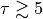.

Parameters
• surface (surface instance) – The extracted emission surface.

• side (optional[str]) – The emission side to plot, must be either 'both', 'front' or 'back'.

• reflect (optional[bool]) – Whether to reflect the back side of the disk about the midplane. Default is False.

• masked (optional[bool]) – Whether to plot the masked points, the default, or all extracted points.

• ax (optional[axes instance]) – The Matplolib axis to use for plotting. If none is provided, one will be generated. If an axis is provided, the same color scaling will be used.

• return_fig (optional[bool]) – If no axis is provided, whether to return the Matplotlib figure. The axis can then be accessed through fig.axes.

Returns

If return_fig=True, the Matplotlib figure used for plotting.

## surface

The surface class is returned from the get_emission_surface() function and was not designed to be created by a user (hence the rather long list of variables required to instantiate the class).

class disksurf.surface(r_f, z_f, I_f, T_f, v, x, y_n, y_f, r_b, z_b, I_b, T_b, y_n_b, y_f_b, chans, rms, x0, y0, inc, PA, r_min, r_max, data)

A container for the emission surface returned by detect_peaks. This class has been designed to be created by the get_emission_surface function and not by the user.

Parameters
• r_f (array) – Radial position of the front surface in [arcsec].

• z_f (array) – Vertical position of the front surface in [arcsec].

• I_f (array) – Intensity along the front surface in [Jy/beam].

• T_f (array) – Brightness temperature along the front surface in [K].

• v (array) – Velocity in [km/s].

• x (array) – Distance along the major axis the point was extracted in [arcsec].

• y_n (array) – Distance along the minor axis of the near peak for the front surface in [arcsec].

• y_f (array) – Distance along the minor axis of the far peak for the front surface in [arcsec].

• r_b (array) – Radial position of the back surface in [arcsec].

• z_b (array) – Vertical position of the back surface in [arcsec].

• I_b (array) – Intensity along the back surface in [Jy/beam].

• T_b (array) – Brightness temperature along the back surface in [K].

• y_n_b (array) – Distance along the minor axis of the near peak for the back surface in [arcsec].

• y_f_b (array) – Distance along the minor axis of the far peak for the back surface in [arcsec].

• chans (tuple) – A tuple of the first and last channels used for the emission surface extraction.

• rms (float) – Noise in the cube in [Jy/beam].

• x0 (float) – Right ascencion offset used in the emission surface extraction in [arcsec].

• y0 (float) – Declination offset used in the emission surface extraction in [arcsec].

• inc (float) – Inclination of the disk used in the emission surface extraction in [deg].

• PA (float) – Position angle of the disk used in the emission surface extraction in [deg].

• r_min (float) – Minimum disk-centric radius used in the emission surface extraction in [arcsec].

• r_max (array) – Maximum disk-centric radius used in the emission surface extraction in [arcsec].

• data (array) – The data used to extract the emission surface in [Jy/beam].

Parameters
• side (optional[str]) – Side of the disk. Must be 'front', 'back' or 'both'. Defaults to 'both'.

• masked (optional[bool]) – Whether to return only the masked points, the default, or all points.

Returns

Vertical cylindrical coordinate in [arcsec].

Parameters
• side (optional[str]) – Side of the disk. Must be 'front', 'back' or 'both'. Defaults to 'both'.

• reflect (optional[bool]) – Whether to reflect the backside points about the midplane. Defaults to False.

• masked (optional[bool]) – Whether to return only the masked points, the default, or all points.

Returns

Vertical cylindrical coordinate in [arcsec].

Intensity at the (r, z) coordinate in [Jy/beam].

Parameters
• side (optional[str]) – Side of the disk. Must be 'front', 'back' or 'both'. Defaults to 'both'.

• masked (optional[bool]) – Whether to return only the masked points, the default, or all points.

Returns

Intensity at the (r, z) coordinate in [Jy/beam].

Brightness temperature at the (r, z) coordinate in [K].

Parameters
• side (optional[str]) – Side of the disk. Must be 'front', 'back' or 'both'. Defaults to 'both'.

• masked (optional[bool]) – Whether to return only the masked points, the default, or all points.

Returns

Brightness temperature at the (r, z) coordinate in [K].

Velocity that the (r, z) coordinate was extracted at in [m/s].

Parameters
• side (optional[str]) – Side of the disk. Must be 'front', 'back' or 'both'. Defaults to 'both'.

• masked (optional[bool]) – Whether to return only the masked points, the default, or all points.

Returns

Velocity that the (r, z) coordinate was extracted at in [m/s].

RA offset that the (r, z) coordinate was extracted in [arcsec].

Parameters
• side (optional[str]) – Side of the disk. Must be 'front', 'back' or 'both'. Defaults to 'both'.

• masked (optional[bool]) – Whether to return only the masked points, the default, or all points.

Returns

RA offset that the (r, z) coordinate was extracted in [arcsec].

Dec offset that the (r, z) coordinate was extracted in [arcsec].

Parameters
• side (optional[str]) – Side of the disk. Must be 'front', 'back' or 'both'. Defaults to 'both'.

• edge (optional[str]) – Which of the edges to return, either the 'near' or 'far' edge.

• masked (optional[bool]) – Whether to return only the masked points, the default, or all points.

Returns

Dec offset that the (r, z) coordinate was extracted in [arcsec].

Inverse aspect ratio (height divided by radius) of the emission surface.

Parameters
• side (optional[str]) – Side of the disk. Must be 'front', 'back' or 'both'. Defaults to 'both'.

• reflect (optional[bool]) – Whether to reflect the backside points about the midplane. Defaults to False.

• masked (optional[bool]) – Whether to return only the masked points, the default, or all points.

Returns

Inverse aspect ratio of the emission surface.

Signal-to-noise ratio for each coordinate.

Parameters
• side (optional[str]) – Side of the disk. Must be 'front', 'back' or 'both'. Defaults to 'both'.

• masked (optional[bool]) – Whether to return only the masked points, the default, or all points.

Returns

Signal-to-noise ratio for each coordinate.

Parameters

side (optional[str]) – Side of the disk. Must be 'front', 'back' or 'both'. Defaults to 'both'.

mask_surface(side='front', reflect=False, min_r=None, max_r=None, min_z=None, max_z=None, min_zr=None, max_zr=None, min_I=None, max_I=None, min_v=None, max_v=None, min_SNR=None, max_SNR=None, RMS=None)

Mask the surface based on simple cuts to the parameters.

Parameters
• min_r (optional[float]) – Minimum radius in [arcsec].

• max_r (optional[float]) – Maximum radius in [arcsec].

• min_z (optional[float]) – Minimum emission height in [arcsec].

• max_z (optional[float]) – Maximum emission height in [arcsec].

• min_zr (optional[float]) – Minimum z/r ratio.

• max_zr (optional[float]) – Maximum z/r ratio.

• min_Inu (optional[float]) – Minumum intensity in [Jy/beam].

• max_Inu (optional[float]) – Maximum intensity in [Jy/beam].

• min_v (optional[float]) – Minimum velocity in [m/s].

• max_v (optional[float]) – Maximum velocity in [m/s].

• min_snr (optional[float]) – Minimum SNR ratio.

• max_snr (optional[float]) – Maximum SNR ratio.

• RMS (optional[float]) – Use this RMS value in place of the self.rms value for calculating the SNR masks.

Bin the emisison surface onto a regular grid. This is a simple wrapper to the binned_parameter function.

Parameters
• rvals (optional[array]) – Desired bin centers.

• rbins (optional[array]) – Desired bin edges.

• side (optional[str]) – Which ‘side’ of the disk to bin, must be one of 'both'’, 'front' or 'back'.

• reflect (Optional[bool]) – Whether to reflect the emission height of the back side of the disk about the midplane.

• masked (Optional[bool]) – Whether to use the masked data points. Default is True.

Returns

The bin centers, r, and the average emission surface, z, with the uncertainty, dz, given as the bin standard deviation.

binned_parameter(p, rvals=None, rbins=None, side='front', reflect=True, masked=True)

Bin the provided parameter onto a regular grid. If neither rvals nor rbins is specified, will default to 50 bins across the radial range of the bins.

Parameters
• p (str) – Parameter to bin. For example, to bin the emission height, p='z'.

• rvals (optional[array]) – Desired bin centers.

• rbins (optional[array]) – Desired bin edges.

• side (optional[str]) – Which ‘side’ of the disk to bin, must be one of 'both'’, 'front' or 'back'.

• reflect (optional[bool]) – Whether to reflect the emission height of the back side of the disk about the midplane.

• masked (optional[bool]) – Whether to use the masked data points. Default is True.

Returns

The bin centers, r, and the binned mean, mu, and standard deviation, std, of the desired parameter.

Return the rolling average of the emission surface. As the radial sampling is unevenly spaced the kernel size, which is a fixed number of samples, can vary in the radial range it represents. The uncertainty is taken as the rolling standard deviation.

Parameters
• window (optional[float]) – Window size in [arcsec].

• side (optional[str]) – Which ‘side’ of the disk to bin, must be one of 'both'’, 'front' or 'back'.

• reflect (optional[bool]) – Whether to reflect the emission height of the back side of the disk about the midplane.

• masked (optional[bool]) – Whether to use the masked data points. Default is True.

Returns

The radius, r, emission height, z, and uncertainty, dz.

rolling_statistic(p, func=numpy.nanmean, window=0.1, side='front', reflect=True, masked=True, remove_NaN=True)

Return the rolling statistic of the provided parameter. As the radial sampling is unevenly spaced the kernel size, which is a fixed number of samples, can vary in the radial range it represents.

Parameters
• p (str) – Parameter to appl the rolling statistic to. For example, to use the emission height, p='z'.

• func (Optional[callable]) – The function to apply to the data.

• window (Optional[float]) – Window size in [arcsec].

• side (Optional[str]) – Which ‘side’ of the disk to bin, must be one of 'both'’, 'front' or 'back'.

• reflect (Optional[bool]) – Whether to reflect the emission height of the back side of the disk about the midplane.

• masked (Optional[bool]) – Whether to use the masked data points. Default is True.

• remove_NaN (Optional[bool]) – Whether to remove the NaNs.

Returns

The radius, r and the rolling statistic, s. All NaNs will have been removed.

fit_emission_surface(tapered_powerlaw=True, include_cavity=False, r0=None, dist=None, side='front', masked=True, return_model=False, curve_fit_kwargs=None)

Fit the extracted emission surface with a tapered power law of the form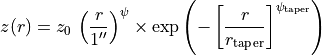where a single power law profile is recovered when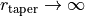, and can be forced using the tapered_powerlaw=False argument.

We additionally allow for an inner cavity,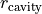, inside which all emission heights are set to zero, and the radial range is shifted such that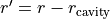. This can be toggled with the include_cavity argument.

The fitting is performed with scipy.optimize.curve_fit where the returned uncertainties are the square root of the diagnal components of the covariance maxtrix returned by curve_fit. We use the SNR of each point as a weighting in the fit.

Parameters
• tapered_powerlaw (optional[bool]) – If True, fit the tapered power law profile rather than a single power law function.

• include_cavity (optional[bool]) – If True, include a cavity in the functional form, inside of which all heights are set to 0.

• r0 (optional[float]) – The reference radius for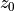. Defaults to 1 arcsec, unless dist is provided, then defaults to 100 au.

• dist (optional[float]) – Convert all distances from [arcsec] to [au] for the fitting. If this is provided, r_ref will change to 100 au unless specified by the user.

• side (optional[str]) – Which ‘side’ of the disk to bin, must be one of 'both'’, 'front' or 'back'.

• masked (optional[bool]) – Whether to use the masked data points. Default is True.

• curve_fit_kwargs (optional[dict]) – Keyword arguments to pass to scipy.optimize.curve_fit.

Returns

Best-fit values, popt, and associated uncertainties, copt, for the fits if return_fit=False, else the best-fit model evaluated at the radial points.

fit_emission_surface_MCMC(r0=None, dist=None, side='front', masked=True, tapered_powerlaw=True, include_cavity=False, p0=None, nwalkers=64, nburnin=1000, nsteps=500, scatter=0.001, priors=None, returns=None, plots=None, curve_fit_kwargs=None, niter=1)

Fit the inferred emission surface with a tapered power law of the form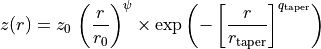where a single power law profile is recovered when, and can be forced using the tapered_powerlaw=False argument.

We additionally allow for an inner cavity,, inside which all emission heights are set to zero, and the radial range is shifted such that. This can be toggled with the include_cavity argument.

The fitting (or more acurately the estimation of the posterior distributions) is performed with emcee. If starting positions are not provided, will use fit_emission_surface to estimate starting positions.

The priors are provided by a dictionary where the keys are the relevant argument names. Each param is described by two values and the type of prior. For a flat prior, priors['name']=[min_val, max_val, 'flat'], while for a Gaussian prior, priors['name']=[mean_val, std_val, 'gaussian'].

Parameters
• r0 (Optional[float]) – The reference radius for. Defaults to 1 arcsec, unless dist is provided, then defaults to 100 au.

• dist (Optional[float]) – Convert all distances from [arcsec] to [au] for the fitting. If this is provided, r_ref will change to 100 au unless specified by the user.

• tapered_powerlaw (optional[bool]) – Whether to include a tapered component to the powerlaw.

• include_cavity (optional[bool]) – Where to include an inner cavity.

• p0 (optional[list]) – Starting guesses for the fit. If nothing is provided, will try to guess from the results of fit_emission_surface.

• nwalkers (optional[int]) – Number of walkers for the MCMC.

• nburnin (optional[int]) – Number of steps to take to burn in.

• nsteps (optional[int]) – Number of steps used to sample the PDF.

• scatter (optional[float]) – Relative scatter used to randomize the starting positions of the walkers.

• priors (optional[dict]) – A dictionary of priors to use for the fitting.

• returns (optional[list]) – A list of properties to return. Can include: 'samples', for the array of PDF samples (default); 'percentiles', for the 16th, 50th and 84th percentiles of the PDF; 'lnprob' for values of the log-probablity for each of the PDF samples; ‘median’ for the median value of the PDFs and 'walkers' for the walkers.

• plots (optional[list]) – A list of plots to make, including 'corner' for the standard corner plot, or 'walkers' for the trace of the walkers.

• curve_fit_kwargs (optional[dict]) – Kwargs to pass to scipy.optimize.curve_fit if the p0 values are estimated through fit_emision_surface.

Returns

Dependent on the returns argument.

plot_surface(ax=None, side='both', reflect=False, masked=True, plot_fit=False, tapered_powerlaw=True, include_cavity=False, return_fig=False)

Plot the emission surface.

Parameters
• ax (Optional[Matplotlib axis]) – Axes used for plotting.

• masked (Optional[bool]) – Whether to plot the maske data or not. Default is True.

• side (Optional[str]) – Which emission side to plot, must be 'front', 'back' or 'both'.

• reflect (Optional[bool]) – If plotting the 'back' side of the disk, whether to reflect it about disk midplane.

• tapered_powerlaw (Optional[bool]) – TBD

• include_cavity (Optional[bool]) – TBD

• return_fig (Optional[bool]) – Whether to return the Matplotlib figure if ax=None.

Returns

If return_fig=True, the Matplotlib figure used for plotting.